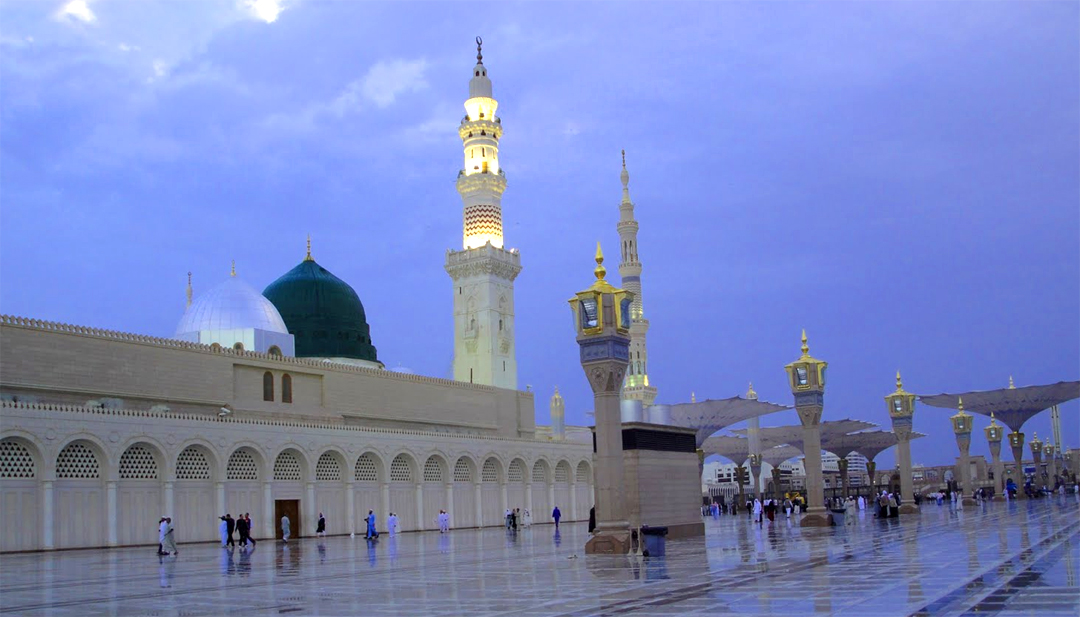5 Questions a day.

20%

Question 1 of 5

1.How many Rukoo (paragraphs) are there in the Quran?

Question 1 of 5

Question 2 of 5

2.How many Manzils (stages) are there in the Quran?

Question 2 of 5

Question 3 of 5

3.How many Surahs (chapters) are there in the Quran?

Question 3 of 5

Question 4 of 5

4.How many Paara or Juz (parts) are there in the Quran?

Question 4 of 5

Question 5 of 5

5.How many Madani Surahs (chapters) are there in the Quran?

Question 5 of 5

Category: